##### Pre-Calculus: 1001 Practice Problems For Dummies (+ Free Online Practice)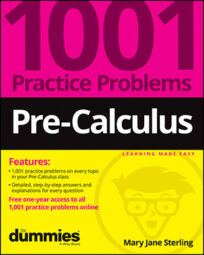In mathematics, a limit suggests that you’re approaching some value. Some functions, such as a rational function with a horizontal asymptote, have a limit as the x values move toward positive or negative infinity — that is, as the value of x gets very small or very large. Limits are another way of describing the characteristics of particular functions.

Although limits are often demonstrated graphically (a picture is worth a thousand words?), you can describe limits more precisely using algebra.

Coupled with limits is the concept of continuity — whether a function is defined for all real numbers or not.

You’ll work on limits and continuity in the following ways:

• Looking at graphs for information on a function’s limits

• Using analytic techniques to investigate limits

• Performing algebraic operations to solve for a function’s limits

• Determining where a function is continuous

• Searching for any removable discontinuities

When you’re working with limits and continuity, some challenges include the following:

• Recognizing a function’s behavior at negative infinity or positive infinity

• Using the correct technique for an analytic look at limits

• Factoring correctly when investigating limits algebraically

• Using the correct conjugates in algebraic procedures

• Forgetting that the “removable” part of a removable discontinuity doesn’t really change a function’s continuity; a function with a removable discontinuity is not continuous

## Practice problems

1. Given the graph of f(x), find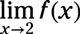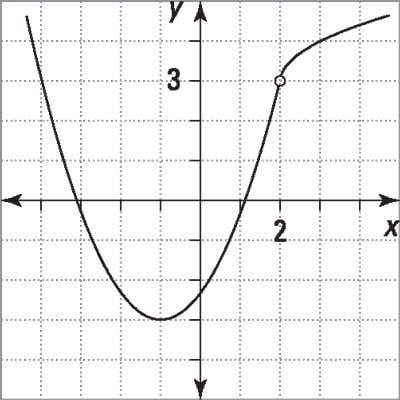Credit: Illustration by Thomson Digital

The function has a hole at (2, 3). The limit as x approaches 2 from the left is 3, and the limit as x approaches 2 from the right is 3.

2. Determine the limit using the values given in the chart: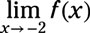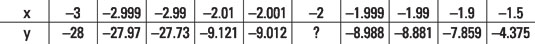Credit: Illustration by Thomson Digital